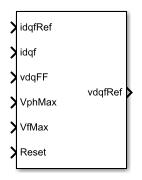# SM Current Controller

Discrete-time synchronous machine current PI controller

• Library:
• Simscape / Electrical / Control / SM Control

•## Description

The SM Current Controller block implements a discrete time PI-based synchronous machine (SM) current controller in the rotor d-q reference frame.

### Defining Equations

The block is discretized using the backward Euler method due to its first-order simplicity and its stability.

Three PI current controllers implemented in the rotor reference frame produce the reference voltage vector:

`${v}_{d}^{ref}=\left({K}_{p_id}+{K}_{i_id}\frac{{T}_{s}z}{z-1}\right)\left({i}_{d}^{ref}-{i}_{d}\right)+{v}_{d_FF},$`

`${v}_{q}^{ref}=\left({K}_{p_iq}+{K}_{i_iq}\frac{{T}_{s}z}{z-1}\right)\left({i}_{q}^{ref}-{i}_{q}\right)+{v}_{q_FF},$`

and

`${v}_{f}^{ref}=\left({K}_{p_if}+{K}_{i_if}\frac{{T}_{s}z}{z-1}\right)\left({i}_{f}^{ref}-{i}_{f}\right),$`

where:

• ${v}_{d}^{ref}$, ${v}_{q}^{ref}$, and ${v}_{f}^{ref}$ are the d-axis, q-axis, and field reference voltages, respectively.

• ${i}_{d}^{ref}$, ${v}_{q}^{ref}$, and ${i}_{f}^{ref}$ are the d-axis, q-axis, and field reference currents, respectively.

• ${i}_{d}$, ${i}_{q}$, and ${i}_{f}$ are the d-axis, q-axis, and field currents, respectively.

• Kp_id, Kp_iq, and Kp_if are the proportional gains for the d-axis, q-axis and field controllers, respectively.

• Ki_id, Ki_iq, and Ki_if are the integral gains for the d-axis, q-axis and field controllers, respectively.

• vd_FF, and vq_FF are the feedforward voltages for the d-axis and q-axis, respectively, obtained from the machine mathematical equations and provided as inputs.

• Ts, is the sample time of the discrete controller.

Using PI control results in a zero in the closed-loop transfer function which can be canceled by introducing a zero-cancelation block in the feedforward path. The zero cancellation transfer functions in discrete time are:

`${G}_{ZC_id}\left(z\right)=\frac{\frac{{T}_{s}{K}_{i_id}}{{K}_{p_id}}}{z+\left(\frac{{T}_{s}-\frac{{K}_{p_id}}{{K}_{i_id}}}{\frac{{K}_{p_id}}{{K}_{i_id}}}\right)},$`

`${G}_{ZC_iq}\left(z\right)=\frac{\frac{{T}_{s}{K}_{i_iq}}{{K}_{p_iq}}}{z+\left(\frac{{T}_{s}-\frac{{K}_{p_iq}}{{K}_{i_iq}}}{\frac{{K}_{p_iq}}{{K}_{i_iq}}}\right)},$`

and

`${G}_{ZC_if}\left(z\right)=\frac{\frac{{T}_{s}{K}_{i_if}}{{K}_{p_if}}}{z+\left(\frac{{T}_{s}-\frac{{K}_{p_if}}{{K}_{i_if}}}{\frac{{K}_{p_if}}{{K}_{i_if}}}\right)}.$`

Saturation must be imposed when the stator voltage vector exceeds the voltage phase limit Vph_max:

`$\sqrt{{v}_{d}^{2}+{v}_{q}^{2}}\le {V}_{ph_max},$`

where vd, and vq are the d-axis and q-axis voltages, respectively.

In the case of axis prioritization, the voltages v1 and v2 are introduced, where:

• v1 = vd and v2 = vq for d-axis prioritization.

• v1 = vq and v2 = vd for q-axis prioritization.

The constrained (saturated) voltages ${v}_{1}^{sat}$ and ${v}_{2}^{sat}$ are obtained as follows:

`${v}_{1}^{sat}=\text{min}\left(\mathrm{max}\left({v}_{1}^{unsat},-{V}_{ph_max}\right),{V}_{ph_max}\right),$`

and

`${v}_{2}^{sat}=\text{min}\left(\mathrm{max}\left({v}_{2}^{unsat},-{V}_{2_max}\right),{V}_{2_max}\right),$`

where:

• ${v}_{1}^{unsat}$ and ${v}_{2}^{unsat}$ are the unconstrained (unsaturated) voltages.

• v2_max is the maximum value of v2 that does not exceed the voltage phase limit, given by ${v}_{2_max}=\sqrt{{\left({V}_{ph_max}\right)}^{2}-{\left({v}_{1}^{sat}\right)}^{2}}.$

In the case that the direct and quadrature axes have the same priority (d-q equivalence) the constrained voltages are obtained as follows:

`${v}_{d}^{sat}=\text{min}\left(\mathrm{max}\left({v}_{d}^{unsat},-{V}_{d_max}\right),{V}_{d_max}\right),$`

and

`${v}_{q}^{sat}=\text{min}\left(\mathrm{max}\left({v}_{q}^{unsat},-{V}_{q_max}\right),{V}_{q_max}\right),$`

where

`${V}_{d_max}=\frac{{V}_{ph_max}|{v}_{d}^{unsat}|}{\sqrt{{\left({v}_{d}^{unsat}\right)}^{2}+{\left({v}_{q}^{unsat}\right)}^{2}}},$`

and

`${V}_{q_max}=\frac{{V}_{ph_max}|{v}_{q}^{unsat}|}{\sqrt{{\left({v}_{d}^{unsat}\right)}^{2}+{\left({v}_{q}^{unsat}\right)}^{2}}}.$`

The constrained (saturated) field voltage ${v}_{f}^{sat}$ is limited according to the maximum admissible value:

`${v}_{f}^{sat}=\text{min}\left(\mathrm{max}\left({v}_{f}^{unsat},-{V}_{f_max}\right),{V}_{f_max}\right),$`

where:

• ${v}_{f}^{unsat}$ is the unconstrained (unsaturated) field voltage.

• Vf_max is the maximum allowable field voltage.

An anti-windup mechanism is employed to avoid saturation of integrator output. In such a situation, the integrator gains become:

`${K}_{i_id}+{K}_{aw_id}\left({v}_{d}^{sat}-{v}_{d}^{unsat}\right),$`

`${K}_{i_iq}+{K}_{aw_iq}\left({v}_{q}^{sat}-{v}_{q}^{unsat}\right),$`

and

`${K}_{i_if}+{K}_{aw_if}\left({v}_{f}^{sat}-{v}_{f}^{unsat}\right),$`

where Kaw_id, Kaw_iq, and Kaw_if are the anti-windup gains for the d-axis, q-axis and field controllers, respectively.

### Assumptions

• The plant model for direct and quadrature axis can be approximated with a first order system.

• This control solution is used only for synchronous motors with sinusoidal flux distribution and field windings.

## Ports

### Input

expand all

Reference d-q and field currents for control of synchronous motor.

Data Types: `single` | `double`

Actual d-q and field axis currents of controlled synchronous motor.

Data Types: `single` | `double`

Feedforward pre-control voltages.

Data Types: `single` | `double`

Maximum allowable voltage in each phase.

Data Types: `single` | `double`

Maximum allowable field voltage.

Data Types: `single` | `double`

External reset signal (rising edge) for integrators.

Data Types: `single` | `double`

### Output

expand all

Reference d-q and field voltages for control of synchronous motor.

Data Types: `single` | `double`

## Parameters

expand all

General

Time, in s, between consecutive block executions. During execution, the block produces outputs and, if appropriate, updates its internal state. For more information, see What Is Sample Time? and Specify Sample Time.

If this block is inside a triggered subsystem, inherit the sample time by setting this parameter to `-1`. If this block is in a continuous variable-step model, specify the sample time explicitly using a positive scalar.

Specify the discretization sample time when zero-cancellation is active and sample time is set to -1 (e.g., when the block is used inside a triggered subsystem).

Prioritize or maintain ratio between d and q axes when block limits voltage.

Enable or disable zero-cancellation on the feedforward path.

Enable or disable pre-control voltage.

d-q Control

Proportional gain of PI controller used for direct-axis current control.

Integrator gain of PI controller used for direct-axis current control.

Anti-windup gain of PI controller used for direct-axis current control.

Proportional gain of PI controller used for quadrature-axis current control.

Integrator gain of PI controller used for quadrature-axis current control.

Anti-windup gain of PI controller used for quadrature-axis current control.

Field Control

Proportional gain of PI controller used for field current control.

Integrator gain of PI controller used for field current control.

Anti-windup gain of PI controller used for field current control.

 Märgner, M., and W. Hackmann. "Control challenges of an externally excited synchronous machine in an automotive traction drive application." Emobility-Electrical Power Train, 2006, pp. 1-6.